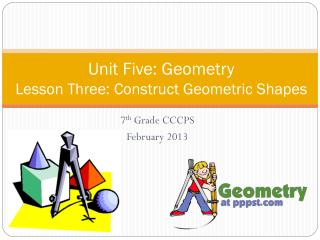Download PresentationUnit Five: Geometry Lesson Three: Construct Geometric Shapes

Unit Five: Geometry Lesson Three: Construct Geometric Shapes - PowerPoint PPT Presentation

Unit Five: Geometry Lesson Three: Construct Geometric Shapes. 7 th Grade CCCPS February 2013. Constructing Triangles. One way to construct triangles is to use computer technology. Another way is to draw it by hand using a ruler, protractor, or both.I am the owner, or an agent authorized to act on behalf of the owner, of the copyrighted work described.
Download PresentationUnit Five: Geometry Lesson Three: Construct Geometric Shapes

Download Policy: Content on the Website is provided to you AS IS for your information and personal use and may not be sold / licensed / shared on other websites without getting consent from its author.While downloading, if for some reason you are not able to download a presentation, the publisher may have deleted the file from their server.

- - - - - - - - - - - - - - - - - - - - - - - - - - E N D - - - - - - - - - - - - - - - - - - - - - - - - - -
Presentation Transcript
1. Unit Five: Geometry Lesson Three: Construct Geometric Shapes 7th Grade CCCPS February 2013

2. Constructing Triangles • One way to construct triangles is to use computer technology. Another way is to draw it by hand using a ruler, protractor, or both. • If you draw it by hand you will be given certain conditions. You can determine if it is possible to draw one unique triangle, more than one triangle (ambiguously defined), or no triangle at all.

3. Constructing Triangles • To determine if triangles are possible, use your two theorems: • Triangle inequality theorem: the sum of the lengths of any two sides of a triangle must be greater than the length of the third side. • You can only use this theorem if you are given side lengths. • Triangle angle sum theorem: the sum of the angles in ANY triangle is 180° • You can only use this theorem if you are given angle measures

4. Is it possible? • Not possible: If it doesn’t pass either of the two theorems • Unique: if you are given three side lengths and they pass the theorem, this triangle can only be drawn once. (If you change the side lengths, its not the same triangle!) • Ambiguously defined: if you are given three angle measures and they pass the theorem, this triangle can be drawn an infinite number of times, just with different side lengths (similar triangles)

5. Example A B F G E C • Note: These two triangles have the same angle measures and pass the angle sum theorem, but are different sizes. • So, given these angle measures, you can draw an infinite number of triangles that are SIMILAR! 54˚

6. Unique Right triangles • Remember: a right triangle must have one right angle. • The side lengths of a right triangle have a special relationship based on the Pythagorean theorem (more about this in 8th grade). • For a triangle to be a right triangle, its side lengths will be in triples. There are multiple triples, but the common ones are listed below • 3,4,5 (Or any multiple of these numbers) • 5, 12, 13 (Or any multiple of these numbers)

7. Examples Describe the following triangles as ambiguously defined, nonexistent, a unique acute triangle, a unique right triangle, or a unique obtuse triangle • A triangle with angles measuring 35°, 85°, and 60°? • A triangle with side lengths of 2inches, 2 inches, and 1 inch • A triangle with side lengths of 6cm, 8cm, and 10cm • A triangle with angles measuring 45°, 90°, and 50°

8. Examples • If Mr. Jordan wants to construct a triangle with angles measuring 67° and 42°, what would have to be the measure of the third angle in his construction? • If Mrs. Lashley constructs a triangle with angles 44°, 23°, and 113°. If she tries to construct a different triangle with those angle measures, what will she discover? • Mrs. Radcliff constructs a triangle with one side 6cm and another side 9cm long. Which is not a possible length for her third side? a. 10cm b. 2cm c. 7cm d. 8cm